## Sphere-Sphere IntersectionLet two spheres of Radiiandbe located along the x-Axis centered atand, respectively. Not surprisingly, the analysis is very similar to the case of the Circle-Circle Intersection. The equations of the two Spheres are(1)(2)

Combining (1) and (2) gives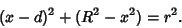(3)

Multiplying through and rearranging give(4)

Solving forgives(5)

The intersection of the Spheres is therefore a curve lying in a Plane parallel to the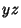-plane at a single-coordinate. Plugging this back into (1) gives(6)

which is a Circle with Radius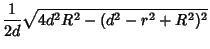(7)

The Volume of the 3-D Lens common to the two spheres can be found by adding the two Spherical Caps. The distances from the Spheres' centers to the bases of the caps are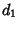(8)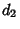(9)

so the heights of the caps are(10)(11)

The Volume of a Spherical Cap of heightfor a Sphere of Radius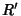is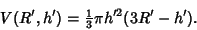(12)

Letting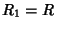and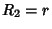and summing the two caps gives(13)

This expression givesfor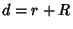as it must. In the special case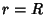, the Volume simplifies to(14)